Tribhuvan University

Institute of Science and Technology

2080

Bachelor Level / second-semester / Science

Computer Science and Information Technology( MTH163 )

Mathematics II

Full Marks: 80

Pass Marks: 32

Time: 3 Hours

Candidates are required to give their answers in their own words as far as practicable.

The figures in the margin indicate full marks.

Group A

Attempt any three questions.

1

Define system of linear equations. When a system of equations is consistent? Make echelon form to solve:

-2a – 3b + 4c = 5

b – 2c = 4

a + 3b – c = 2

2

Define linear transformation with an example.

Let A =, v =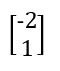, b=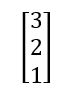, x =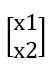,

and define a transformation T : R² → R² by T(x) = Ax then

a. find T(v).

b. Find x ∈ R² whose image under T is b.

3

Find AB by block multiplication of the matrices.

A=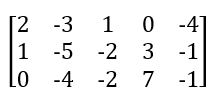B=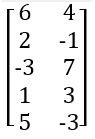4

Find the least square solution of Ax=c where

A=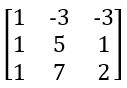, c=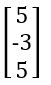and compute the associated least square error.

Group B

Attempt any ten questions.

5

Determine the column of the matrix A are linearly independent where

A =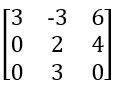6

Let A =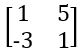and B =. What value (s) of k, if any, will make AB=BA?

7

Evaluate the determinant of the matrix.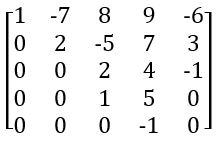8

When two column vectors in R² are equal? Give an example. Compute u+3v, -u-2v where,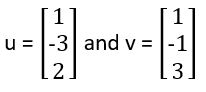9

Prove that the two vectors u and v are perpendicular to each other if and only if the line through u is perpendicular bisector of the line segment from -u to v.

10

Find the eigenvalue of A =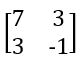11

Define null space of a matrix A. Letthen show that v belongs to the null space matrix A.

12

Find the equation y = a0 + a1 x of the least squares line that best fits the data points (2,1), (5,2), (7,3), (8,3).

13

Show that the solution of yk+2 – 4yk+1 + 3yk = 0 are linearly independent.

14

Define group. Show that the set of integers is a group with respect to addition operation.

15
Define ring and show that set of positive integers with respect to addition and multiplication operation is not a ring.